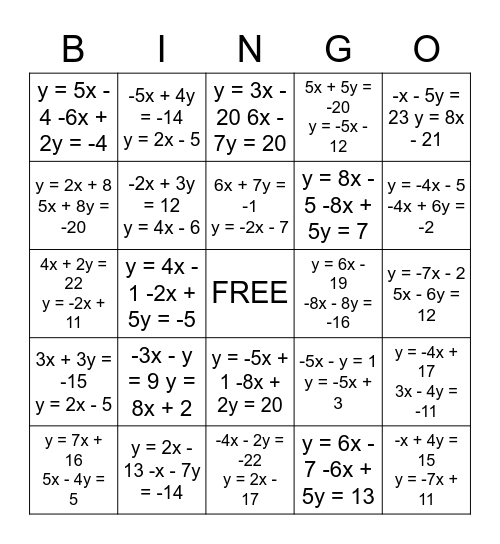# Substitution BingoThis bingo card has a free space and 25 words: -3x - y = 9 y = 8x + 2, -4x - 2y = -22 y = 2x - 17, 6x + 7y = -1 y = -2x - 7, y = 7x + 16 5x - 4y = 5, -x - 5y = 23 y = 8x - 21, y = 5x - 4 -6x + 2y = -4, y = 2x + 8 5x + 8y = -20, -5x + 4y = -14 y = 2x - 5, y = 6x - 19 -8x - 8y = -16, y = -5x + 1 -8x + 2y = 20, y = 8x - 5 -8x + 5y = 7, 4x + 2y = 22 y = -2x + 11, -5x - y = 1 y = -5x + 3, y = -4x + 17 3x - 4y = -11, -2x + 3y = 12 y = 4x - 6, 6x + 7y = 15 y = -5x - 2, y = -7x - 2 5x - 6y = 12, y = 3x - 20 6x - 7y = 20, y = -4x - 5 -4x + 6y = -2, y = 4x - 1 -2x + 5y = -5, -x + 4y = 15 y = -7x + 11, y = 2x - 13 -x - 7y = -14, 3x + 3y = -15 y = 2x - 5, 5x + 5y = -20 y = -5x - 12 and y = 6x - 7 -6x + 5y = 13.

## Play Online